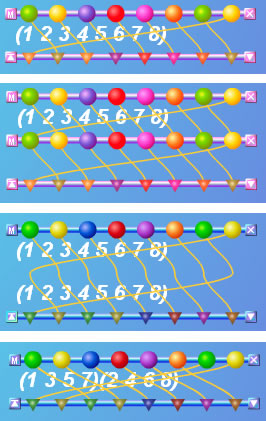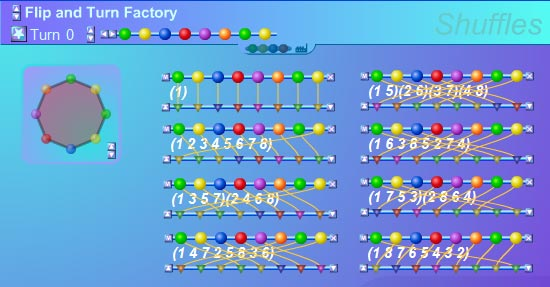#### You may also like### DOTS Division

Take any pair of two digit numbers x=ab and y=cd where, without loss of generality, ab > cd . Form two 4 digit numbers r=abcd and s=cdab and calculate: {r^2 - s^2} /{x^2 - y^2}.### Common Divisor

Find the largest integer which divides every member of the following sequence: 1^5-1, 2^5-2, 3^5-3, ... n^5-n.### Hypotenuse Lattice Points

The triangle OMN has vertices on the axes with whole number co-ordinates. How many points with whole number coordinates are there on the hypotenuse MN?

# Zodiac

##### Age 14 to 16 Challenge Level:

In Sheffuls , you will find an introduction to the cycle notation which you'll need to use for this investigation. The Shuffles interactivity will also help. We're going to investigate turns within turns. Watch out for those Zodiacal alignments!

Let's start by generating all the permutations that can be made by repeating the cycle $(1 2 3 4 5 6 7 8)$ which we'll call $t_8$.

In the Shuffles interactivity you can do this by choosing the length 8 in the Flip and Turn Factory. Pull down two copies of Turn 1 from the menu bar and compose them to obtain Turn 2, as illustrated in the diagram below:There is also a short tutorial that explains how to create and manipulate shuffles.

Compose Turn 2 with another copy of Turn 1 and you will have Turn 3. Keep going, and after tidying things up a bit you should have all eight of them:You can see how these shuffles relate to turns of the octagon by pressing the bottom right play buttons.

In cycle notation you can make Turn 2 like this: $$t_8^2 = (1 2 3 4 5 6 7 8)(1 2 3 4 5 6 7 8) = (1 3 5 7)(2 4 6 8).$$ Think '1 goes to 2, and then 2 goes to 3; so 1 goes to 3'.

Then Turn 3 = $t_8^3 = (1 3 5 7)(2 4 6 8)(1 2 3 4 5 6 7 8)$ and so on.

You should end up with a similar table like this:

$$\begin{eqnarray} t^0 &=& (1)\\ t_8^1 &=& (1 2 3 4 5 6 7 8)\\ t_8^2 &=& (1 3 5 7)(2 4 6 8)\\ t_8^3 &=& (1 4 7 2 5 8 3 6)\\ t_8^4 &=& (1 5)(2 6)(3 7)(4 8)\\ t_8^5 &=& (1 6 3 8 5 2 7 4)\\ t_8^6 &=& (1 7 5 3)(2 8 6 4)\\ t_8^7 &=& (1 8 7 6 5 4 3 2) \end{eqnarray}$$

Notice that $t_8^2$ contains two cycles, but $t_8^3$ contains just one, and $t_8^4$ contains 4. Why might this be?

Investigate what happens with length 9 and length 10 cycles. As a check, you should find that $t_9^3$ contains 3 cycles, but $t_9^2$ contains just one.

Can you find a general rule? How many cycles will $t_{23}^2$ contain? How many cycles will $t_{30}^{10}$ contain?

Now look at Stars . Notice anything?